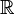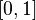# Uncountable set facts for kids

Kids Encyclopedia Facts

An uncountable set is an infinite set that is impossible to count. If we try to count the elements, we will always skip some. It does not matter what size step we take. The set of real numbers, often written as$\mathbb{R}$, is an uncountable set. There are many other uncountable sets, such as the interval$[0, 1]$. An uncountable set is bigger than an infinite countable set. We know that because Georg Cantor proved it. He showed that any list of numbers is not complete. This is true even if the list is infinite.

## Related pagesUncountable set Facts for Kids. Kiddle Encyclopedia.